# Recursion Collections API Recursion Revisited Programming Assignments using

• Slides: 23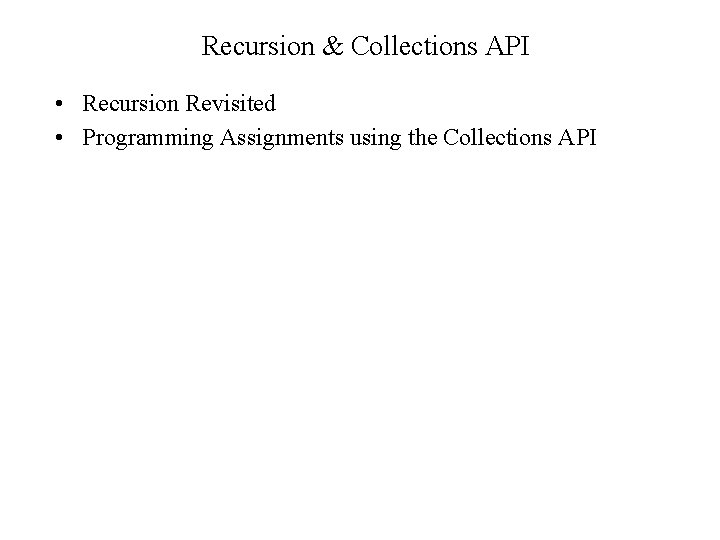Recursion & Collections API • Recursion Revisited • Programming Assignments using the Collections API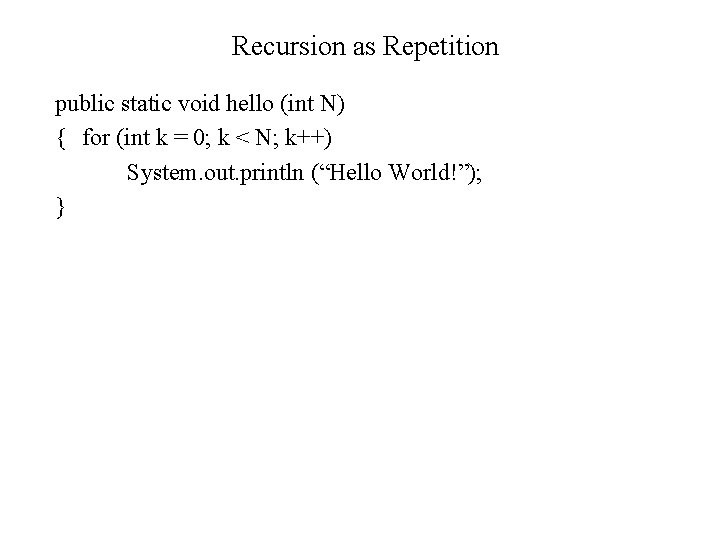Recursion as Repetition public static void hello (int N) { for (int k = 0; k < N; k++) System. out. println (“Hello World!”); }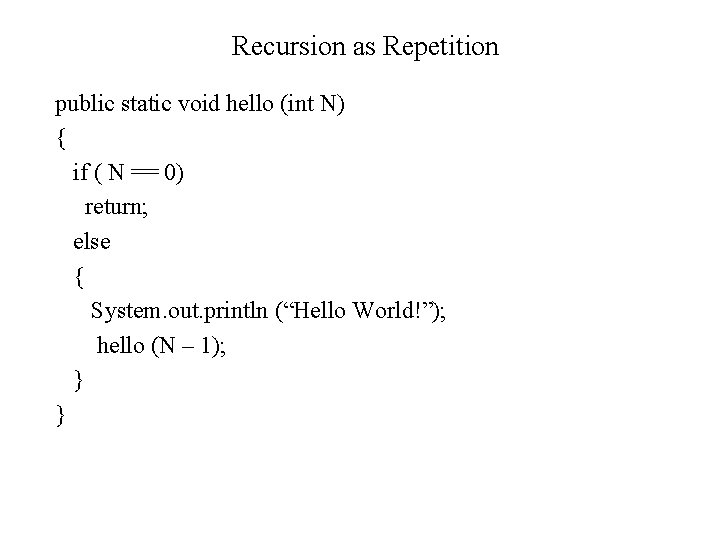Recursion as Repetition public static void hello (int N) { if ( N == 0) return; else { System. out. println (“Hello World!”); hello (N – 1); } }Recursion as Repetition • Write a recursive method pow() that returns xn, where x and n are both integers greater than or equal to zero.Recursion as Repetition public static long pow (int x, int n) { if ( x == 0) return 0; if ( n == 0) return 1; long result = x * pow ( x, n – 1); return result; }Recursive String Methods • Write a recursive method that will print the characters in a string recursively, one character at a time.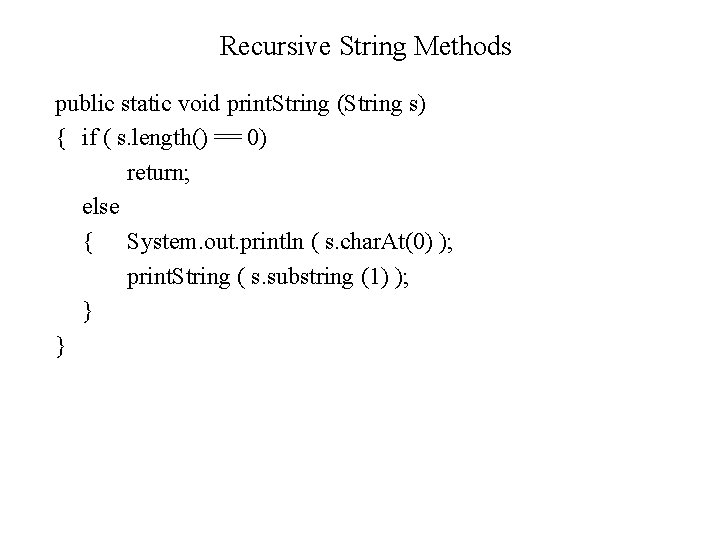Recursive String Methods public static void print. String (String s) { if ( s. length() == 0) return; else { System. out. println ( s. char. At(0) ); print. String ( s. substring (1) ); } }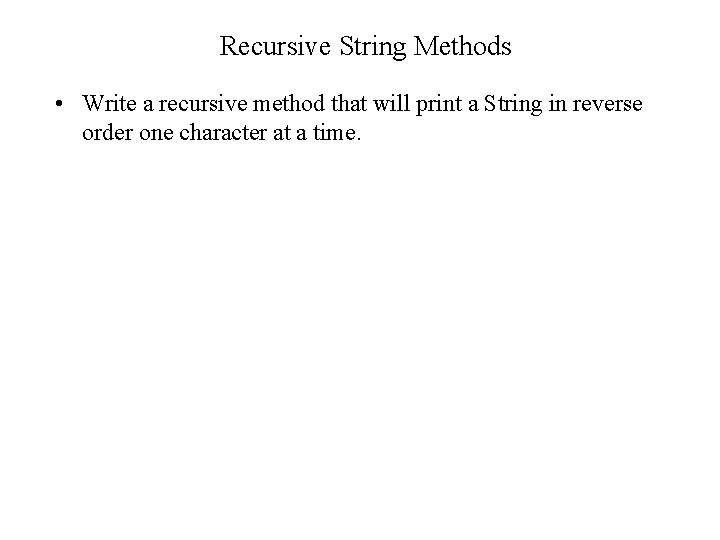Recursive String Methods • Write a recursive method that will print a String in reverse order one character at a time.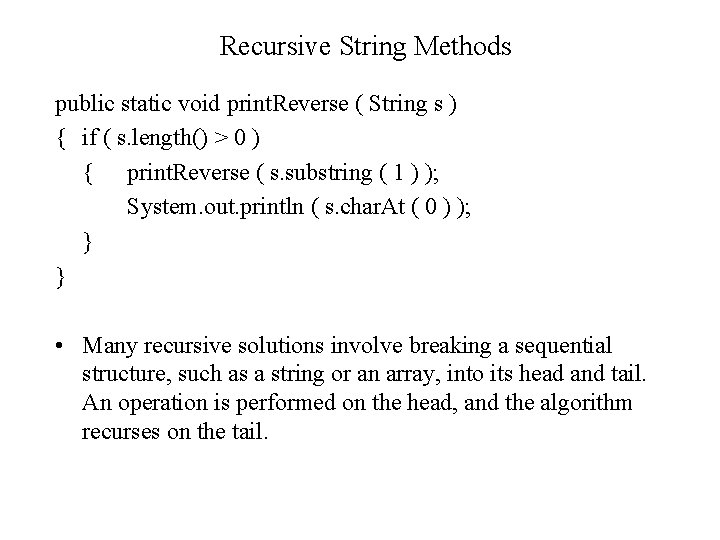Recursive String Methods public static void print. Reverse ( String s ) { if ( s. length() > 0 ) { print. Reverse ( s. substring ( 1 ) ); System. out. println ( s. char. At ( 0 ) ); } } • Many recursive solutions involve breaking a sequential structure, such as a string or an array, into its head and tail. An operation is performed on the head, and the algorithm recurses on the tail.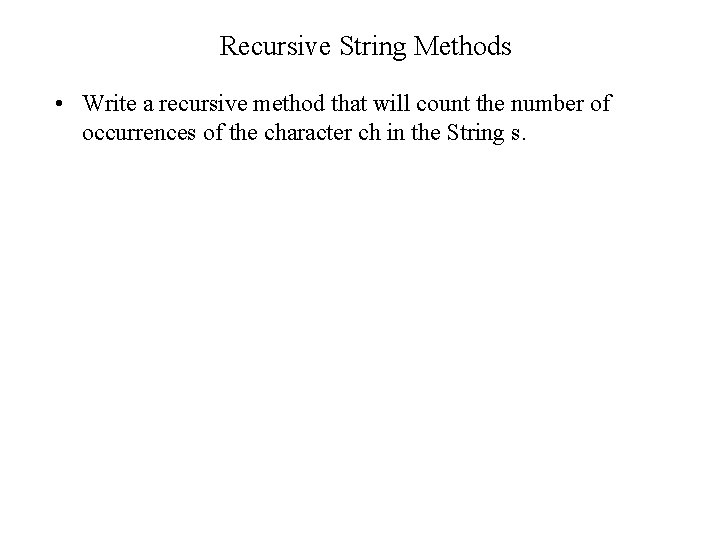Recursive String Methods • Write a recursive method that will count the number of occurrences of the character ch in the String s.Recursive String Methods public static int count. Char (String s, char ch) { if ( s. length() == 0 ) return 0; else if ( s. char. At ( 0 ) == ch) return 1 + count. Char ( s. substring (1), ch); else return 0 + count. Char ( s. substring (1), ch); }Recursive String Methods • Write a recursive method to rotate a String by N characters to the right. For example, rotate. R (“hello”, 3) should return “llohe”.Recursive String Methods public static String rotate. R (String s, int n) { if ( n == 0 ) return s; else { String. Buffer buf = new String. Buffer (); buf. append (s. char. At (s. length() - 1)); buf. append (s. substring (0, s. length() - 1)); return rotate. R (buf. to. String(), n - 1); } }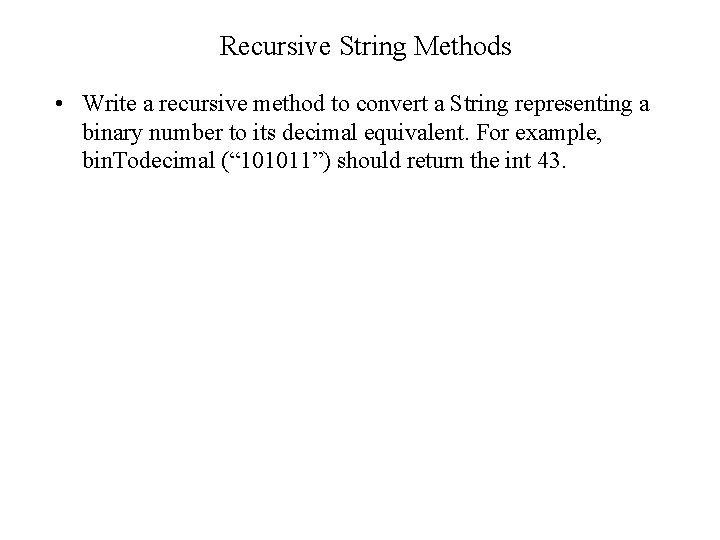Recursive String Methods • Write a recursive method to convert a String representing a binary number to its decimal equivalent. For example, bin. Todecimal (“ 101011”) should return the int 43.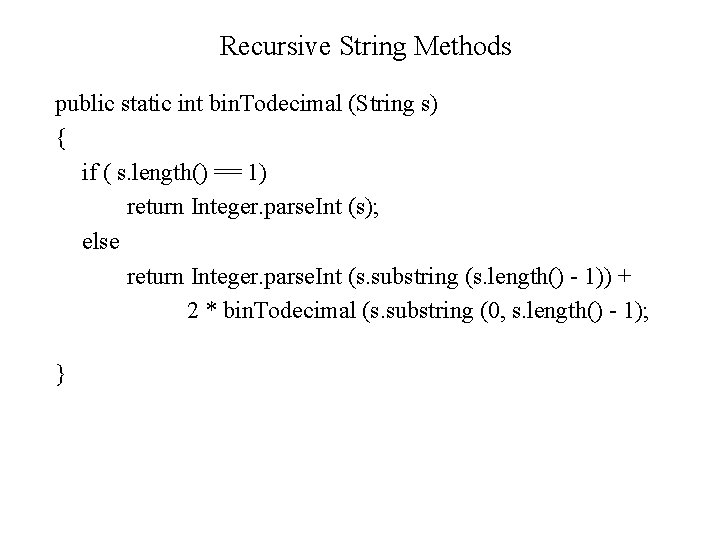Recursive String Methods public static int bin. Todecimal (String s) { if ( s. length() == 1) return Integer. parse. Int (s); else return Integer. parse. Int (s. substring (s. length() - 1)) + 2 * bin. Todecimal (s. substring (0, s. length() - 1); }Recursive String Methods • A palindrome is a string that is the same as its reverse – “radar” and “able was I ere I saw elba”. Write a recursive boolean method that determines whether its String parameter is a palindrome.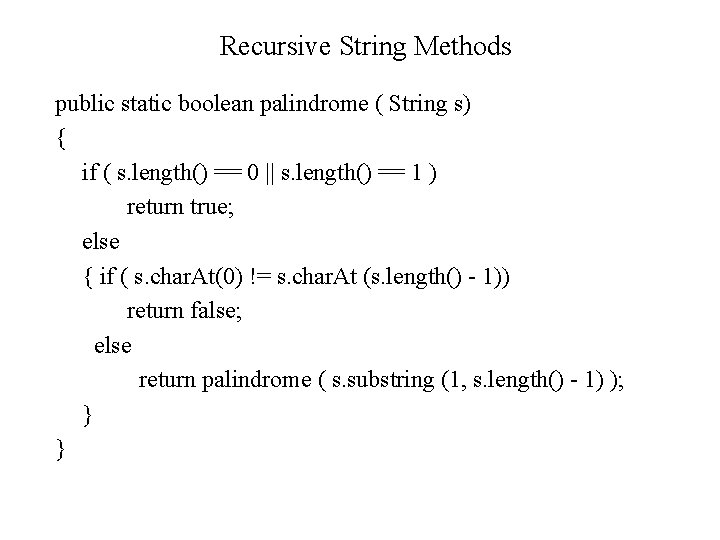Recursive String Methods public static boolean palindrome ( String s) { if ( s. length() == 0 || s. length() == 1 ) return true; else { if ( s. char. At(0) != s. char. At (s. length() - 1)) return false; else return palindrome ( s. substring (1, s. length() - 1) ); } }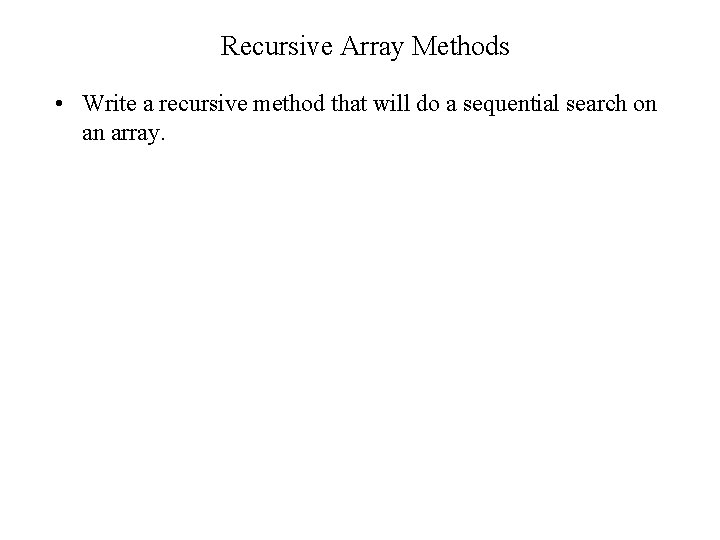Recursive Array Methods • Write a recursive method that will do a sequential search on an array.Recursive Array Methods public static int r. Search (int[] arr, int head, int key ) { if ( head == arr. length ) return – 1; else if ( arr[head] == key ) return head; else return r. Search ( arr, head + 1, key ); }Recursive Array Methods • Write a recursive method that will do a selection sort on an array.Recursive Array Methods public static void selection. Sort ( int[] arr, int last ) { if ( last > 0 ) { int max. Loc = find. Max. Idx ( arr, last); swap ( arr, last, max. Loc ); selection. Sort ( arr, last – 1 ); } } public static int find. Max. Idx ( arr, last) { int max. Idx = 0; for (int i = 0; i <= last; i++) if ( arr [i] > arr[max. Idx) max. Idx = i; }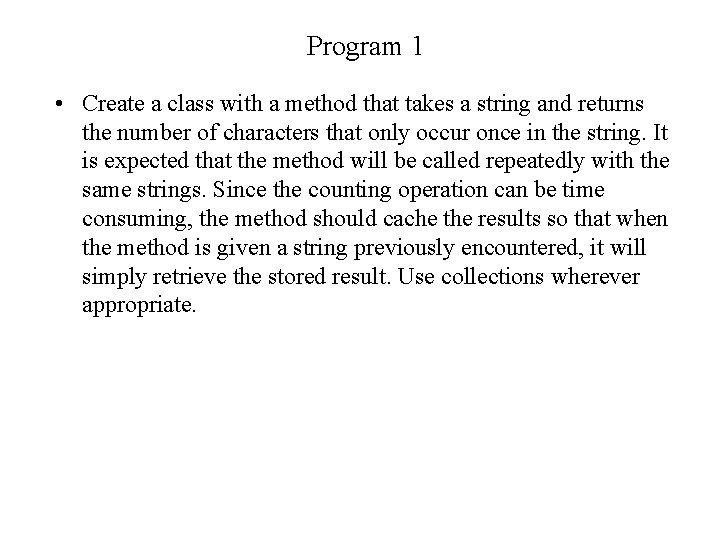Program 1 • Create a class with a method that takes a string and returns the number of characters that only occur once in the string. It is expected that the method will be called repeatedly with the same strings. Since the counting operation can be time consuming, the method should cache the results so that when the method is given a string previously encountered, it will simply retrieve the stored result. Use collections wherever appropriate.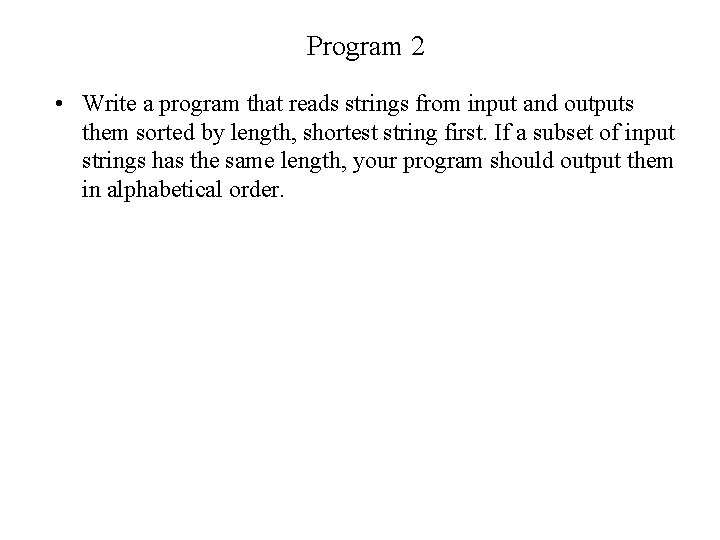Program 2 • Write a program that reads strings from input and outputs them sorted by length, shortest string first. If a subset of input strings has the same length, your program should output them in alphabetical order.